Study of a boat hull shape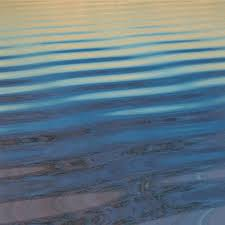The study of the forces of resistance on a boat hull shape allows to evaluate the propulsive force that will serve to advance to the desired speed. The force resistance to forward (expressed in Newtons) is opposed to the ship. This force may be measured by towing the vessel. Propulsive force equal and opposite will be provided by the propulsion machinery to maintain the speed of the ship. As it is not possible to tow a ship that is not yet built, this it is necessary to formulate a practical and accessible method giving the designer and shipbuilder concrete design elements of the propulsion machinery.
It is convenient to decompose the total hydrodynamic force resistance (Rh), distinguishing three main resistances causing the total resistance:
1. Viscous resistance (Rv) composed of
• Frictional resistance dependent on the wetted surface and roughness .
• resistance pressure dependent on the hull shape and swirls it generates
2. La résistance de vagues (Rw) dépendant de la vitesse et de la longueur du navire..
3. The aerodynamic resistance (Ra) superstructures exposed to the wind (which will be considered here as negligible)
We owe this idea Froude study Separate the viscous resistance (Rv), from the wave resistance (Rw) and aerodynamic resistance (Ra). The separation of forces composing the total resistance may seem questionable because it interact with each other (the formation of a wave along the hull shape changes the wetted surface and hence the frictional resistance). But if we take care to assess this interaction distinguishing different wave regimes, it provides us with useful results to draw the hulls of boats.
There is a relationship between the length and between two crests of a wave (L meters) and velocity (V m / sec) given by::
• V/(9.81 X L)=0.4
which can be formulated: (V/0.4)²/9.81=L or: L = (2Pi /9.81) x V²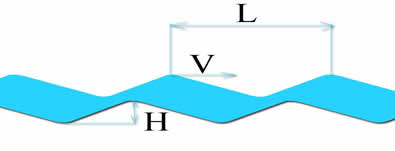Incidentally, as it is possible to determine the energy contained in a wave by the equation: E (joules) = (1/8) x density (kg/m3) x 9.81 x H ² x L, we could estimate energy absorbed by the waves observed..
:
• The waves created by the ship have the same speed as him, so it is possible to determine the relation: L = (2Pi /9.81) x V² the distance between two crests of the waves produced by the boat Replacing V by speed boat..
• Substituting in the relation V/(9.81 X L)=0.4 , The wave length of by the waterline length Lf, Froude deduce the significant number of wave effects on the resistance to the advancement of ships, and the formula: C/(9.81 X Lf) designated the Froude number with C = speed of the boat and Lf = waterline length. Froude number Fn allows us to assess the shape of the waves accompanying the ship. Evaluation of the wave regime and evolution of specific resistance as a function of Froude number: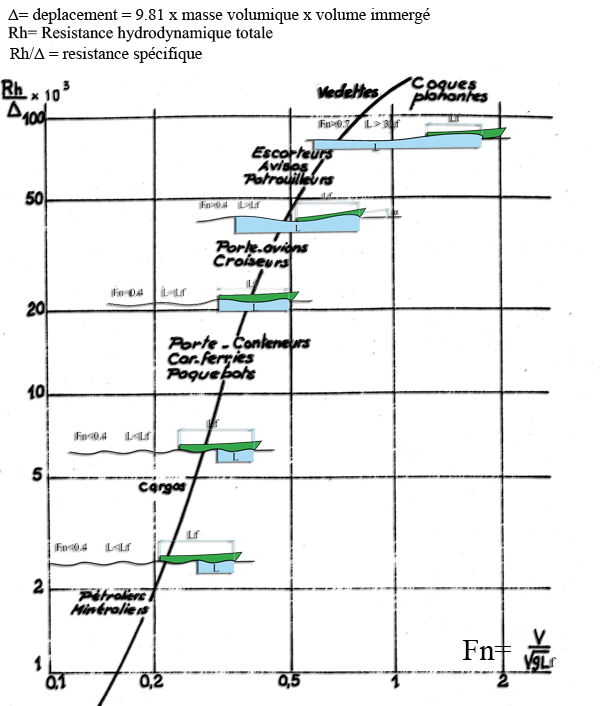See in detail the remarkable values ​​of the Froude number and impactt:

Froude number less than 0.4: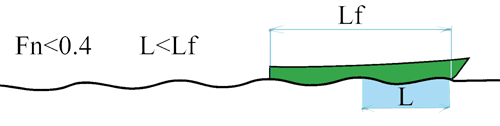• At low Froude number (lower is 0.26) 3 ridges low height at least along the boat. The wave resistance is low and the resistance of friction represents 70 to 90% of the resistance to advancement. Above 0.26 resistance wave increases and the interaction between the Bow wave and the wave occurs REAR to significant way on the resistance to advancement by the effect of adding or subtracting the height of ridges. See in more detail the interaction:
The ship generates mainly two wave trains :
1. Waves emerging in the Bow and with a maximum height of the first peak is a 1/4 of the length L
2. Waves created by sucking the rear of the boat, emerging at the back of the boat and starting with a hollow
We noted earlier that it is possible to determine the energy contained in a wave by the equation: E (joules) = (1/8) x density (kg/m3) ² x 9.81 x H x L, we can estimate that the wave resistance will be strong if system wave generated by the displacement of a hollow (H) important. The combination or cancellation of waves thus indicates peaks or troughs in the wave resistance:• If Lf is a multiple of L on a crest of wave Bow which overlaps and cancels the hollow of the emerging system at the rear.'s Resistance to the advancement through a minimum
• If Lf is odd multiple of L / 2 (eg Lf X1 = L / 2 or 3 x Lf = L / 2), then the wave outcome of the Bow present a hollow at the rear of the ship that combines with wave REAR and resistance to the advancement through a maximum.
These values ​​correspond to a remarkable series of Froude number: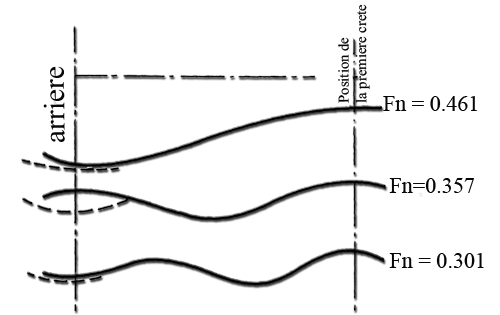That we can place on a curve of wave resistance: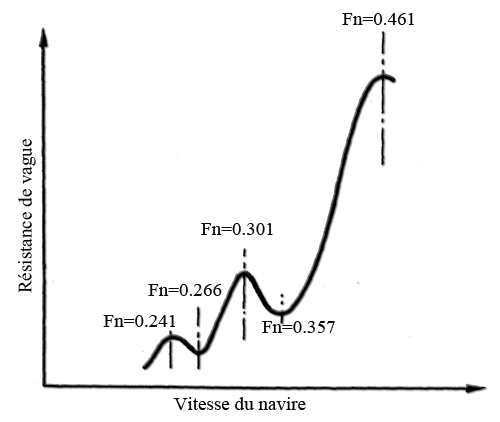Froude number = 0.4: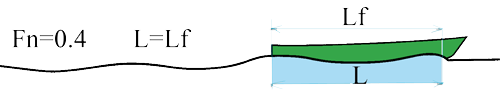The wavelength corresponds to the length of the hull shape, the wave REAR is canceled by the bow wave. This is the theoretical maximum speed for which the hull shape was designed. The vessel is in its wave, a crest on the front, a ridge at the back and the hollow in the middle. The speed increase is accompanied by a sharp increase in wave resistance:

Froude number > 0.4 :Froude number between 0.4 and 0.5 The ship goes on the crest of the bow while the hollows approaching the back. angle appears. This is the worst situation and the most costly in wave resistance which can reach 85% of the total resistance to progress. The ship presents a surface area increased by angle. The resistance curve rises to a maximum arrow has Fn = 0.461

Froude number > 0.5: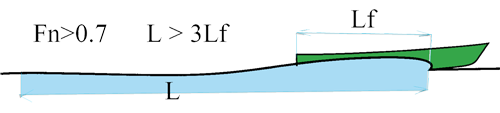The ship is on the crest wave of bow and clears the bow. while finding a horizontal position. This is the area of lightweight planing hulls equipping ships. A flat surface on the back is scheduled to take support on the wave without the press and hold the maximum hull out of the water. The wave resistance decreases sharply.

Overall methodology for calculating resistance to the advancement of hulls.Consider a plane and infinite calm water. We neglect the aerodynamic resistance. Method of calculationl:
• Calculation of the wet surface
• Calculating the ship displacement
• Calculating the number of froude
• Estimated wave regime
• Estimating the angle of camber of the vessel
• Estimated residual drag by the method of Savitsky
• Calculation of the viscous resistance: calculating the frictional resistance + resistance calculation pressure (form)